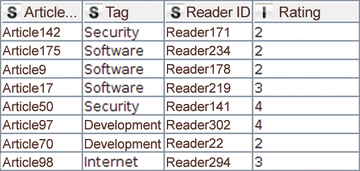# Analyze This!

#### Opening a Connection

The next step is to relate the imported data to each other. If you are familiar with databases, you know that the best way to do this is with a join. A join consists of one or more predicates that determine which rows belong together in two tables. The rows that belong together are then combined in an output table.

This example needs to combine the two tables based on the article ID. In this case, the join predicate determines that ratings for an article must be linked to the categories to which the same article is assigned. The node that KNIME uses for this is Joiner . Its output table is shown in Figure 4. Like its database counterpart, Joiner supports various modes, including inner, outer, left, and right joins. The user can also select which columns are to be copied from which table into the output.Figure 4: Output table for the Joiner node.

#### Pivoting

The next step is to create a kind of fingerprint for each reader, describing the reader's preferences by calculating the reader's average rating for the articles in each category.

The result is a table in which each reader becomes a row and each category becomes a column. The cells of the intersection points display the reader's average rating for articles in each category (Figure 5).

This transformation is handled by the Pivoting node. The Pivoting node dialog may prove intimidating at first glance, since it requires information on the group, pivot, and aggregation columns in three separate tabs. The values in the group columns determine which rows need to be combined into a single row – that is, all those that have the same values in these columns. For the sample workflow, the reader ID is a group column, the category is the pivot column, and the aggregation column contains the ratings that an average function averages.

If a reader has not rated a single article for a category, the cell in the pivotized table does not contain a value. KNIME indicates this with a red question mark. You can replace missing values using the Missing Value node. In the example, it makes sense to assume that the reader does not like a category in which they have never rated an article. Instead of the missing value, the table will use a zero.

It is often the case that a node produces a table with long or incomprehensible column names. For example, the Pivoting node from the previous category creates columns in the format Category + Rating . The suffix, which is the same in each column, is not important. Two important KNIME nodes assist with renaming columns. Column Rename lets you rename each column name explicitly; Column Rename (Regex) lets you change the column names using regular expressions.

#### Cluster Analysis

Now that the data is transformed into a workable format, you can begin to group readers according to their preferences. Readers with similar preferences should end up in the same group. Cluster analysis is a useful technique for this kind of problem, and KNIME has different nodes to support it, including k-means, fuzzy c-means, hierarchical agglomerative clustering, or k-medoids.

What all these cluster algorithms have in common is that users have to decide for themselves how many groups they want to receive. They also all need a way to calculate the similarity between two datapoints. In the previous category, a table row was created for each reader, containing five numeric values – one for each category.

The workflow for the example uses the k-Means node, which is quite fast and generally produces good results. The algorithm works with different distance measurements – in KNIME it is implemented with the Euclidean distance (see the "Computing the Distance" box). The k-Means node in KNIME, which executes the algorithm, appends another column to the input table that remembers the group in which the datapoint lies.

Computing the Distance

Let each reader be a five-component vector consisting of the ratings in the five categories. The following formula then computes the distance between the two readers `x` and `y`:

The k-means algorithm initiates the following steps to find homogeneous groups:

1. Initialize the cluster centers with random datapoints.

2. Compute the nearest cluster center for each datapoint.

3. Compute the center for each group of datapoints assigned to a center in Step 2.

4. Update the cluster centers with the newly found centers.

5. If the centers remain unchanged, stop the computations; otherwise go to step 2.

Cluster analysis reveals that readers who give a high rating to articles in the categories Hardware and Security usually lie in cluster 0, while readers from cluster 1 prefer the categories Internet and Software, and cluster 2 prefers Development and Software. Once you know the results, it is possible to give the clusters meaningful names. Due to their preferences, for example, readers in Cluster 0 can be addressed as administrators, those in Cluster 1 as power users, and those in Cluster 2 as developers. These new names are added using the Cell Replacer node.

## Buy this article as PDF

Express-Checkout as PDF
Price \$2.95
(incl. VAT)

SINGLE ISSUES

SUBSCRIPTIONS

## Related content

comments powered by Disqus